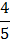# If the ratio of the concentration of electron to that of holes in a semiconductor isand the ratio of current is, then what is the ratio of their drift velocities a)b)c)d)## Question ID - 150265 :- If the ratio of the concentration of electron to that of holes in a semiconductor isand the ratio of current is, then what is the ratio of their drift velocities a)b)c)d)3537

 (b)